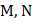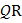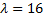#is a square andare the midpoints of the sidesand, respectively. If the ratio of the areas of the square and the triangleis, thenis equal to a) 2 b) 4 c) 2 d) 16

## Question ID - 53539 :-is a square andare the midpoints of the sidesand, respectively. If the ratio of the areas of the square and the triangleis, thenis equal to a) 2 b) 4 c) 2 d) 16

3537

(b)

We can assume thatandare-axis and-axis, respectively. Let. Then area of squareisCoordinates ofandareandrespectivelyNext Question :
 Consider 3 lines as fallows0If these lines enclose a triangleand sum of the squares of the tangent to the interior angles can be expressed in the formwhereandare relatively prime numbers, then the value ofis a) 500 b) 450 c) 230 d) 465